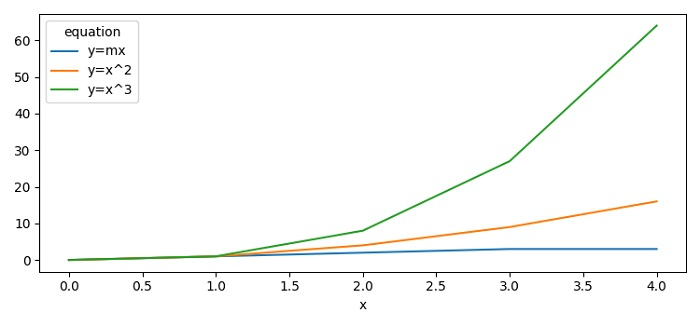# Plotting multiple line graphs using Pandas and Matplotlib

To plot multiple line graphs using Pandas and Matplotlib, we can take the following steps −

• Set the figure size and adjust the padding between and around the subplots.

• Make a 2D potentially heterogeneous tabular data using Pandas DataFrame class, where the column are x, y and equation.

• Get the reshaped dataframe organized by the given index such as x, equation, and y.

• Use the plot() method to plot the lines.

• To display the figure, use show() method.

## Example

import pandas as pd
from matplotlib import pyplot as plt
plt.rcParams["figure.figsize"] = [7.50, 3.50]
plt.rcParams["figure.autolayout"] = True
df = pd.DataFrame([
["y=x^3", 0, 0],
["y=x^3", 1, 1],
["y=x^3", 2, 8],
["y=x^3", 3, 27],
["y=x^3", 4, 64],
["y=x^2", 0, 0],
["y=x^2", 1, 1],
["y=x^2", 2, 4],
["y=x^2", 3, 9],
["y=x^2", 4, 16],
["y=mx", 0, 0],
["y=mx", 1, 1],
["y=mx", 2, 2],
["y=mx", 3, 3],
["y=mx", 4, 3],
], columns=['equation', 'x', 'y'])
df = df.pivot(index='x', columns='equation', values='y')
df.plot()
plt.show()

## Output